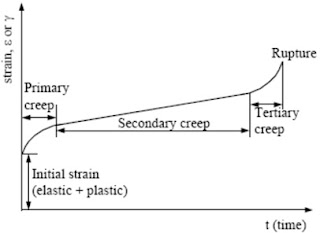### Mechanics of Solids - short answer questions from AMIE exams (Winter 2018)

Explain the following in brief  (2 marks each)

### Free body diagram.

In many problems, it is essential to isolate the body under consideration from the other bodies in contact and draw all the forces acting on the body. For this, first, the body is drawn and then applied
forces, self-weight and the reactions at the points of contact with other bodies are drawn. Such a
diagram of the body in which the body under consideration is freed from all the contact surfaces and
shows all the forces acting on it (including reactions at contact surfaces), is called a Free Body Diagram (FBD).

### Relationship between elastic constants.Where,
K is the Bulk modulus
G is the shear modulus or modulus of rigidity.
E is Young’s modulus or modulus of Elasticity.
Unit of Modulus of elasticity E is N/m² or Pascal.

### Mohr’s circle.

Mohr’s circle is a graphical method of finding normal, tangential and resultant stresses on an oblique plane. Mohr’s circle will be drawn for the following cases :
• A body is subjected to two mutually perpendicular principal tensile stresses of unequal intensities.
• A body is subjected to two mutually perpendicular principal stresses which are unequal and unlike (i.e., one is tensile and the other is compressive).
• A body is subjected to two mutually perpendicular principal tensile stresses accompanied by simple shear stress.
Consider a rectangular body subjected to two mutually perpendicular principal tensile stresses of unequal intensities. It is required to find the resultant stress on an oblique plane.
Let σ1 = Major tensile stress; σ2 = Minor tensile stress, and θ = Angle made by the oblique plane with the axis of minor tensile stress.

Then Mohr circle would be like this:

### Creep curve.

Creep is the tendency of a solid material to move slowly or deform permanently under the influence of persistent mechanical stresses. It can occur as a result of long-term exposure to high levels of stress that are still below the yield strength of the material. Creep is more severe in materials that are subjected to heat for long periods and generally increases as they near their melting point.A creep curve

A creep curve usually consists of three portions corresponding to particular stages of creep.
• The first stage is the stage of what is called unstable, or transient creep (also called initial or primary creep). It features a gradual decrease of the deformation rate to a definite constant value.
• The second stage is the stage of steady-state, or secondary creep and is characterized by a constant deformation rate. At the third stage (tertiary, or accelerated creep), the deformation rate increases up to failure.
• The third stage is, as a rule, short and should be avoided, since the quick failure of parts is inevitable at this stage.

### Stress-strain-temperature relations.

Thermal stresses are the stresses induced in a body due to changes in temperature. Thermal stresses are set up in a body when the temperature of the body is raised or lowered and the body is not allowed to expand or contract freely. But if the body is allowed to expand or contract freely, no stresses will be set up in the body.

Stress = Strain × E = α.T.E

### Difference between thin and thick cylinders.

Thin cylinder
• The cylinder which has a thickness is less than 1/10 to 1/20 of its diameter, that cylinder is called a thin cylinder.
• A cylinder whose wall thickness is less than 1/20 times of its internal diameter
• The thin cylinder is only resisted by internal pressure.
• Low stress consuming capacity.
Thick cylinder
• The cylinder which has a thickness is more than 1/20 of its diameter that cylinder is called a thick Cylinder.
• A cylinder whose wall thickness is greater than 1/20 times its internal diameter.
• The thick cylinder is resisted by internal as well as external pressure.
• More stress consuming capacity.

### Location of maximum radial stress in a thin rotating annular disk.

The equation of maximum radial stress in a thin rotating annular disk is### Distribution of bending stress in rectangular section beam.

Consider following the beam of rectangular section. Bending stress distribution is shown.

The maximum stress (either compressive or tensile) takes place at the outermost layer.

General equation isM = Moment acting at the beam,
R = Radius of curvature of the beam.
y = the distance of the point from the neutral axis.

### Yield criterion

A yield criterion is a hypothesis defining the limit of elasticity in a material and the onset of plastic deformation under any possible combination of stresses. A yield condition or yield criterion describes a material failure in structural plasticity. It defines the threshold state of material between elastic and plastic or brittle failure deformations. It is very important to adopt a proper yield criterion in the design of a structure.

---
The study material for AMIE/B Tech/Junior Engineer exams is available at https://amiestudycircle.com